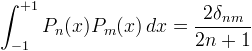﻿OrthogonalPolynomials.LegendreP Method (Int32, Double)Computes the value of a Legendre polynomial.

Namespace:  Meta.Numerics.Functions
Assembly:  Meta.Numerics (in Meta.Numerics.dll) Version: 4.1.4Syntax
```public static double LegendreP(
int l,
double x
)```

#### Parameters

l
Type: SystemInt32
The order, which must be non-negative.
x
Type: SystemDouble
The argument, which must lie on the closed interval between -1 and +1.

#### Return Value

Type: Double
The value of Pl(x).Exceptions
ExceptionCondition
ArgumentOutOfRangeExceptionx lies outside [-1,+1].Remarks

Legendre polynomials are orthogonal on the interval [-1,1].See Also